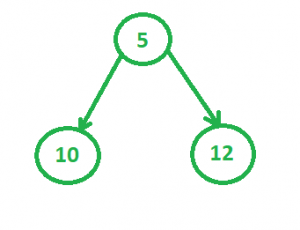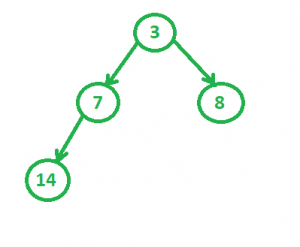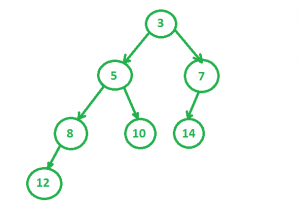# Skew Heap

A skew heap (or self – adjusting heap) is a heap data structure implemented as a binary tree. Skew heaps are advantageous because of their ability to merge more quickly than binary heaps. In contrast with binary heaps, there are no structural constraints, so there is no guarantee that the height of the tree is logarithmic. Only two conditions must be satisfied :

1. The general heap order must be there (root is minimum and same is recursively true for subtrees), but balanced property (all levels must be full except the last) is not required.
2. Main operation in Skew Heaps is Merge. We can implement other operations like insert, extractMin(), etc using Merge only.

Example :
1. Consider the skew heap 1 to be2. The second heap to be considered4. And we obtain the final merged tree asRecursive Merge Process :
merge(h1, h2)

1. Let h1 and h2 be the two min skew heaps to be merged. Let h1’s root be smaller than h2’s root (If not smaller, we can swap to get the same).
2. We swap h1->left and h1->right.
3. h1->left = merge(h2, h1->left)

Examples :

```Let h1 be
10
/    \
20      30
/        /
40        50

Let h2 be
15
/    \
25      35
/  \
45    55

After swapping h1->left and h1->right, we get
10
/    \
30      20
/        /
50        40

Now we recursively Merge
30
/     AND
40

15
/    \
25      35
/  \
45    55
After recursive merge, we get (Please do it
using pen and paper).
15
/    \
30      25
/  \       \
35    40      45

We make this merged tree as left of original
h1 and we get following result.
10
/      \
15        20
/    \      /
30    25    40
/   \     \
35   40    45
```

For visualization : https://www.cs.usfca.edu/~galles/JavascriptVisual/LeftistHeap.html

 `// CPP program to implement Skew Heap ` `// operations. ` `#include ` `using` `namespace` `std; ` ` `  `struct` `SkewHeap ` `{ ` `    ``int` `key; ` `    ``SkewHeap* right; ` `    ``SkewHeap* left; ` ` `  `    ``// constructor to make a new ` `    ``// node of heap ` `    ``SkewHeap() ` `    ``{ ` `        ``key = 0; ` `        ``right = NULL; ` `        ``left = NULL; ` `    ``} ` ` `  `    ``// the special merge function that's ` `    ``// used in most of the other operations ` `    ``// also ` `    ``SkewHeap* merge(SkewHeap* h1, SkewHeap* h2) ` `    ``{ ` `        ``// If one of the heaps is empty ` `        ``if` `(h1 == NULL) ` `            ``return` `h2; ` `        ``if` `(h2 == NULL) ` `            ``return` `h1; ` ` `  `        ``// Make sure that h1 has smaller ` `        ``// key. ` `        ``if` `(h1->key > h2->key) ` `           ``swap(h1, h2); ` ` `  `        ``// Swap h1->left and h1->right ` `        ``swap(h1->left, h1->right); ` ` `  `        ``// Merge h2 and h1->left and make ` `        ``// merged tree as left of h1. ` `        ``h1->left = merge(h2, h1->left); ` ` `  `        ``return` `h1; ` `    ``} ` ` `  `    ``// function to construct heap using ` `    ``// values in the array ` `    ``SkewHeap* construct(SkewHeap* root, ` `                     ``int` `heap[], ``int` `n) ` `    ``{ ` `        ``SkewHeap* temp; ` `        ``for` `(``int` `i = 0; i < n; i++) { ` `            ``temp = ``new` `SkewHeap; ` `            ``temp->key = heap[i]; ` `            ``root = merge(root, temp); ` `        ``} ` `        ``return` `root; ` `    ``} ` ` `  `    ``// fucntion to print the Skew Heap, ` `    ``// as it is in form of a tree so we use ` `    ``// tree traversal algorithms ` `    ``void` `inorder(SkewHeap* root) ` `    ``{ ` `        ``if` `(root == NULL) ` `            ``return``; ` `        ``else` `{ ` `            ``inorder(root->left); ` `            ``cout << root->key << ``"  "``; ` `            ``inorder(root->right); ` `        ``} ` `        ``return``; ` `    ``} ` `}; ` ` `  `// Driver Code ` `int` `main() ` `{ ` `    ``// Construct two heaps ` `    ``SkewHeap heap, *temp1 = NULL, ` `                   ``*temp2 = NULL; ` `    ``/* ` `            ``5 ` `           ``/ \ ` `          ``/   \ ` `         ``10   12    */` `    ``int` `heap1[] = { 12, 5, 10 }; ` `    ``/* ` `            ``3 ` `           ``/ \ ` `          ``/   \ ` `         ``7     8 ` `        ``/ ` `       ``/ ` `      ``14    */` `    ``int` `heap2[] = { 3, 7, 8, 14 }; ` `    ``int` `n1 = ``sizeof``(heap1) / ``sizeof``(heap1); ` `    ``int` `n2 = ``sizeof``(heap2) / ``sizeof``(heap2); ` `    ``temp1 = heap.construct(temp1, heap1, n1); ` `    ``temp2 = heap.construct(temp2, heap2, n2); ` ` `  `    ``// Merge two heaps ` `    ``temp1 = heap.merge(temp1, temp2); ` `    ``/* ` `            ``3 ` `           ``/ \ ` `          ``/   \ ` `         ``5     7 ` `        ``/ \   / ` `       ``8  10 14 ` `      ``/ ` `     ``12    */` `    ``cout << ``"Merged Heap is: "` `<< endl; ` `    ``heap.inorder(temp1); ` `} `

Output:

```The heap obtained after merging is:
12  8  5  10  3  14  7
```

My Personal Notes arrow_drop_upCheck out this Author's contributed articles.

If you like GeeksforGeeks and would like to contribute, you can also write an article using contribute.geeksforgeeks.org or mail your article to contribute@geeksforgeeks.org. See your article appearing on the GeeksforGeeks main page and help other Geeks.

Please Improve this article if you find anything incorrect by clicking on the "Improve Article" button below.

Article Tags :
Practice Tags :

1

Please write to us at contribute@geeksforgeeks.org to report any issue with the above content.